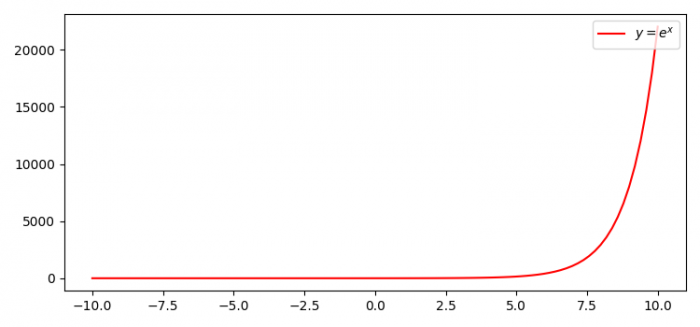# How to write your own LaTeX preamble in Matplotlib?

To write your own LaTeX preamble in Matplotlib, we can take the following steps −

• Set the figure size and adjust the padding between and around the subplots.
• Create x and y random data points using numpy.
• Plot x and y data points using plot() method. Use LaTex formatting for the label.label="$y=e^{x}$"
• Place a legend on the figure using legend() method.
• To display the figure, use show() method.

## Example

import numpy as np
from matplotlib import pyplot as plt

plt.rcParams["figure.figsize"] = [7.50, 3.50]
plt.rcParams["figure.autolayout"] = True

x = np.linspace(-10, 10, 100)
y = np.exp(x)

plt.plot(x, y, color='red', label="$y=e^{x}$")
plt.legend(loc='upper right')

plt.show()

## Output